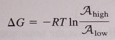# Problem: The synthesis of one ATP molecule requires 2H+ to pass through the phosphorylation enzyme. The difference in free energy when a molecule travels from a region of high activity to a region of low activity is How big must the pH difference be (at 298 K) if the passage of two protons is to provide enough energy to synthesize one ATP molecule?

###### FREE Expert Solution

Recall: Proton-motive force

$\mathbf{\Delta G}\mathbf{=}\mathbf{2}\mathbf{.}\mathbf{3}\mathbf{\left(}\mathbf{n}\mathbf{\right)}\mathbf{RT\Delta pH}$

Synthesis of ATP requires 34.5 kJ/mol.###### Problem Details

The synthesis of one ATP molecule requires 2H+ to pass through the phosphorylation enzyme. The difference in free energy when a molecule travels from a region of high activity to a region of low activity isHow big must the pH difference be (at 298 K) if the passage of two protons is to provide enough energy to synthesize one ATP molecule?